# KS1 Maths Quiz - Year 2 Measurements - Units (Questions)

This quiz addresses the requirements of the National Curriculum KS1 Maths and Numeracy for children aged 6 and 7 in year 2. Specifically this quiz is aimed at the section dealing with choosing and using appropriate standard units to estimate and measure.

Children in Year 1 are exposed to and expected to use the appropriate standard units of measurement for height (cm/m), weight (g/kg), temperature (oC) and capacity (ml/l). This quiz will help them to become familiar with the appropriate units to use for each measurement.

To see a larger image, click on the picture.
1. What units could we use to measure his height?[ ] Kilometres and metres [ ] Litres and millilitres [ ] Kilograms and grams [ ] Metres and centimetres
2. Which unit would we use to measure the amount of water in the bath?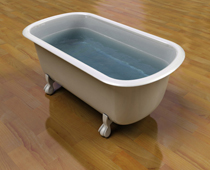[ ] Litres [ ] Centimetres [ ] Grams [ ] Kilometres
3. Which unit would we use to measure one of the tomatoes' weight?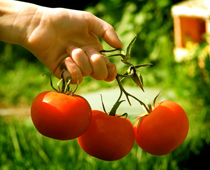[ ] Kilograms [ ] Grams [ ] Millimetres [ ] Litres
4. Which unit would we use to measure the height of a tall building?[ ] Kilograms [ ] Millimetres [ ] Centimetres [ ] Metres
5. Which unit would we use to measure the weight of the cow?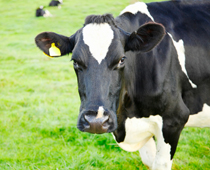[ ] Kilograms [ ] Centimetres [ ] Litres [ ] Metres
6. Which unit would we use to measure the temperature on the island?[ ] Litres [ ] Centimetres [ ] oC [ ] Grams
7. Which unit would we use to measure how much juice is in the glass?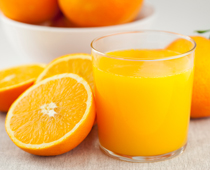[ ] Litres [ ] Millilitres [ ] Centimetres [ ] Grams
8. Which unit would we use to measure the length of the caterpillar?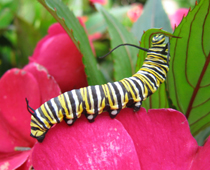[ ] Metres [ ] Centimetres [ ] Litres [ ] Grams
9. Which unit would we use to measure how much water the boy will put in the pool?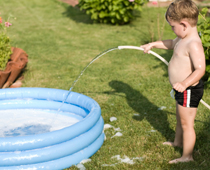[ ] Litres [ ] Grams [ ] Metres [ ] Millilitres
10. Which unit would we use to measure the length of the book?[ ] Centimetres [ ] Metres [ ] Millilitres [ ] GramsKS1 Maths Quiz - Year 2 Measurements - Units (Answers)
1. What units could we use to measure his height?[ ] Kilometres and metres [ ] Litres and millilitres [ ] Kilograms and grams [x] Metres and centimetres
He might be just over a metre tall so we'd need to use centimetres too
2. Which unit would we use to measure the amount of water in the bath?[x] Litres [ ] Centimetres [ ] Grams [ ] Kilometres
Litres are useful for larger amounts of liquid
3. Which unit would we use to measure one of the tomatoes' weight?[ ] Kilograms [x] Grams [ ] Millimetres [ ] Litres
Kilograms wouldn't be any use as the tomato wouldn't be heavy enough
4. Which unit would we use to measure the height of a tall building?[ ] Kilograms [ ] Millimetres [ ] Centimetres [x] Metres
Some tall buildings can be hundreds of metres tall!
5. Which unit would we use to measure the weight of the cow?[x] Kilograms [ ] Centimetres [ ] Litres [ ] Metres
A cow weighs more than 500kg!
6. Which unit would we use to measure the temperature on the island?[ ] Litres [ ] Centimetres [x] oC [ ] Grams
Temperatures are measured in degrees
7. Which unit would we use to measure how much juice is in the glass?[ ] Litres [x] Millilitres [ ] Centimetres [ ] Grams
The juice is less than a litre so we would use millilitres
8. Which unit would we use to measure the length of the caterpillar?[ ] Metres [x] Centimetres [ ] Litres [ ] Grams
This caterpillar is about 7 or 8 centimetres long
9. Which unit would we use to measure how much water the boy will put in the pool?[x] Litres [ ] Grams [ ] Metres [ ] Millilitres
There will be about 100 litres in the pool
10. Which unit would we use to measure the length of the book?[x] Centimetres [ ] Metres [ ] Millilitres [ ] Grams
The book is about 40cm long